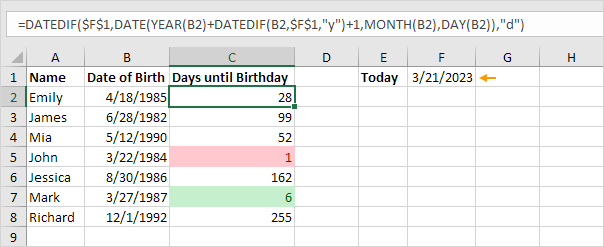# Days until Birthday

To calculate the number of days until your birthday in Excel, execute the following steps.

1. Enter the date of birth into cell B2.2. Enter the TODAY function into cell C2 to return today's date.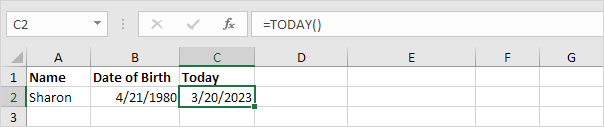3. The most difficult part in order to get the number of days until your birthday is to find your next birthday. The formula below does the trick.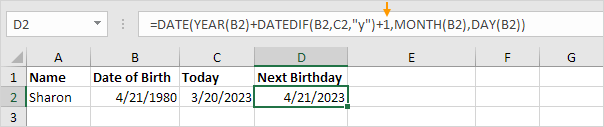Explanation: The DATE function accepts three arguments: year, month and day. We used the DATEDIF function to find the number of complete years ("y") between Date of Birth and Today. In this example, DATEDIF(B2,C2,"y") equals 42. If 42 complete years have passed since your date of birth (in other words, you have already celebrated your 42nd birthday), your next birthday will be 42 + 1 = 43 years after your date of birth.

4. Next, we use the DATEDIF function to find the number of days ("d") between Today and Next Birthday.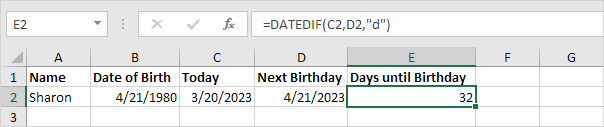Conclusion: Sharon's birthday is in 32 days.

If you have a list of names and birthdates, you can use Excel to highlight upcoming birthdays. This is pretty cool.

5. For example, the formula below calculates how many days are left until Emily's birthday.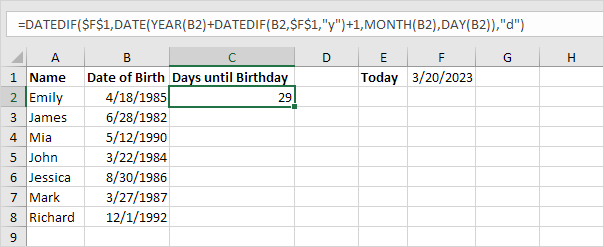Note: this formula isn't new. To create this formula, take the formula from step 4 and replace D2 with the formula from step 3. Next, replace C2 with \$F\$1.

6. Double click the lower right corner of cell C2 to quickly copy the formula to the other cells.7. To highlight upcoming birthdays within a week (green) or within a day (red), you can use conditional formatting in Excel.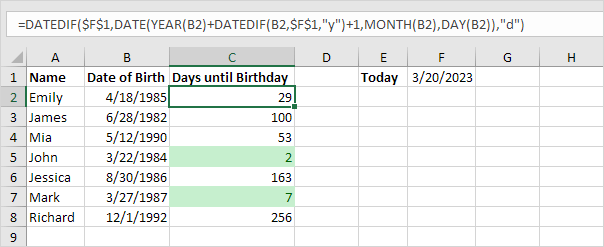8. This is what the spreadsheet will look like tomorrow.